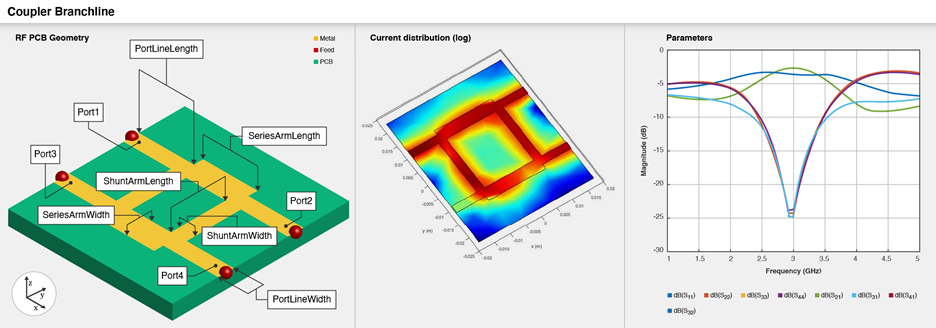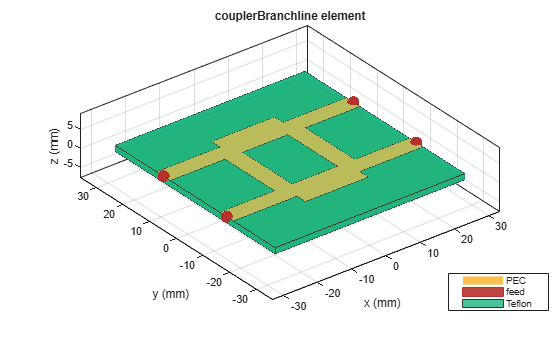# couplerBranchline

Create branch line coupler or quadrature hybrid

## Description

Use the `couplerBranchline` object to create a branch line coupler or a quadrature hybrid. A branch line coupler or a quadrature hybrid divides the power between two ports with a phase difference of 90 degrees. This PCB component has four ports. By default, port 1 is the input port, port 2 is the through port, port 4 is the coupled port, and port 3 is the isolated port.To analyze the behavioral model for the branchline coupler, set the `Behavioral` property in the `sparameters` to `true` or 1.

## Creation

### Syntax

``coupler = couplerBranchline``
``coupler = couplerBranchline(Name=Value)``

### Description

example

````coupler = couplerBranchline` creates a branch line coupler. The default property values are for the frequency of 3 GHz.```
````coupler = couplerBranchline(Name=Value)` sets Properties using one or more name-value arguments. For example, `couplerBranchline(PortLineLength=0.0286)` creates a branch line coupler of length 0.0286 meters. Properties not specified retain their default values.```

## Properties

expand all

Length of the input and the output line in meters, specified as a positive scalar.

Example: `coupler = couplerBranchline(PortLineLength=0.0286)`

Data Types: `double`

Width of the input and the output line in meters, specified as a positive scalar.

Example: `coupler = couplerBranchline(PortLineWidth=0.0070)`

Data Types: `double`

Length of the series arm in meters, specified as a positive scalar.

Example: `coupler = couplerBranchline(SeriesArmLength=0.0286)`

Data Types: `double`

Width of the series arm in meters, specified as a positive scalar.

Example: `coupler = couplerBranchline(SeriesArmWidth=0.0096)`

Data Types: `double`

Length of the shunt arm in meters, specified as a positive scalar.

Example: `coupler = couplerBranchline(ShuntArmLength=0.0286)`

Data Types: `double`

Width of the shunt arm in meters, specified as a positive scalar.

Example: `coupler = couplerBranchline(ShuntArmWidth=0.0096)`

Data Types: `double`

Height of the branch line coupler from the ground plane in meters, specified as a positive scalar.

In the case of a multilayer substrate, you can use the `Height` property to create a branch line coupler line where the two dielectrics interface.

Example: `coupler = couplerBranchline(Height=0.0076)`

Data Types: `double`

Width of the ground plane in meters, specified as a positive scalar.

Example: `coupler = couplerBranchline(GroundPlaneWidth=0.046)`

Example: double

Type of dielectric material used as a substrate, specified as a `dielectric` object.

Example: `d = dielectric("FR4");` `coupler = couplerBranchline(Substrate=d)`

Data Types: `string` | `char`

Type of metal used in the conducting layers, specified as a `metal` object.

Example: `m = metal("PEC");` `coupler = couplerBranchline(Conductor=m)`

Data Types: `string` | `char`

## Object Functions

 `charge` Calculate and plot charge distribution `coupling` Calculate coupling factor of coupler `current` Calculate and plot current distribution `design` Design branchline coupler around particular frequency `directivity` Calculate directivity of coupler `feedCurrent` Calculate current at feed port `getZ0` Calculate characteristic impedance of transmission line `isolation` Calculate isolation of coupler `layout` Plot all metal layers and board shape `mesh` Change and view mesh properties of metal or dielectric in PCB component `shapes` Extract all metal layer shapes of PCB component `show` Display PCB component structure or PCB shape `sparameters` Calculate S-parameters for RF PCB objects

## Examples

collapse all

Create and view a default branchline coupler.

`coupler = couplerBranchline`
```coupler = couplerBranchline with properties: PortLineLength: 0.0186 PortLineWidth: 0.0051 SeriesArmLength: 0.0184 SeriesArmWidth: 0.0083 ShuntArmLength: 0.0186 ShuntArmWidth: 0.0051 Height: 0.0016 GroundPlaneWidth: 0.0600 Substrate: [1x1 dielectric] Conductor: [1x1 metal] ```
`show(coupler)`Pozar, David M. Microwave Engineering. 4th ed. Hoboken, NJ: Wiley, 2012.